Search

Matrix Notation / Types of Matrices (page 2 of 3)

Sections: Augmented & coefficient matrices / Matrix size, Matrix notation & types, Matrix equalityMatrix Notation and Formatting

A note regarding formatting. When you write a matrix, you must use brackets: " [ ] ". Do not use absolute-value bars: " | | ", as they have a different meaning in this context. Do not use parentheses or curly braces ( " { } " ) or some other grouping symbol (or no grouping symbol at all), as these presentations have no meaning. A matrix is always inside square brackets. Use the correct notation, or your answers may be counted as incorrect.

As mentioned earlier, the values contained within a matrix are called "entries". For whatever reason, matrixes are customarily named with capital letters, such as "A" or "B", and the entries are named using the corresponding lower-case letters, but with subscripts. In a matrix A, the entries will typically be named "ai,j", where "i" is the row of A and "j" is the column of A. For instance, given the following matrix A: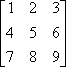...the value 4 is in the second row and the first column, so 4 is the 2,1-entry. That is, 4 = a2,1 (pronounced as "ay-sub-two-one"). The 3,2-entry is the value in the third row and the second column, so a3,2 = 8. Entry a1,3 is 3.   Copyright © Elizabeth Stapel 2003-2011 All Rights Reserved

For smaller matrices (those with fewer than ten rows and columns), the comma in the subscript is sometimes omitted. For instance, "a1,3 = 3" might be written as "a13 = 3". This obviously won't work for larger matrices, since "a213" would be unclear. (Does it indicate the 21,3-entry or the 2,13-entry?) It is probably a good idea, regardless of the notation used in your book, to use commas in your subscripts, for clarity's sake.

Types of matrices

Sometimes matrices are categorized according to the configurations of their entries.

 For instance, a matrix like this one, with all-zero entries below the top-left-to-lower-right diagonal ("the diagonal") is called "upper triangular". (You can have lower triangular matrices, too, but they aren't of much use, so "triangular", without the "upper" or "lower", is generally taken to mean "upper triangular".)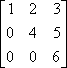A matrix with non-zero entries only on the diagonal is called "diagonal".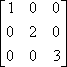A diagonal matrix whose non-zero entries are all 1's is called an "identity" matrix, for reasons which will become clear when you learn how to multiply matrices.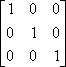There are many identity matrices. The previous example was the 3 × 3 identity; this is the 4 × 4 identity: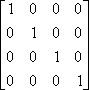The 3 × 3 identity is denoted by I3 (pronounced as "eye-three" or "eye-sub-three"); similarly, the 4 × 4 identity is I4 and the 2 × 2 identity matrix is I2: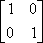Note that triangular matrices are square, that diagonals are triangular and therefore are square, and that identities are diagonals and therefore are triangular and square. When describing a matrix, you usually just give its most specific classification, as this implies all the others.

• Classify the following matrix:
•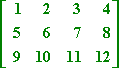This isn't a square matrix, so it can't be an identity or anything. This is just a...

3 × 4 matrix.

• Classify the following matrix:
•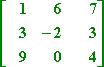This is a square matrix, but there isn't anything else special about it.

3 × 3 square matrix

• Classify the following matrix:
•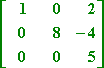This is a 3 × 3 upper triangular matrix, but it is not diagonal.

3 × 3 upper triangular matrix

Note: In a triangular matrix, you can have additional zeroes on or above the diagonal.

• Classify the following matrix:
•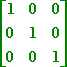This is a diagonal matrix, and, more than that, the diagonal entries are all 1's. Then this is...

the 3 × 3 identity, I3.

Since identity matrices are, by definition, square matrices, you only need to use one subscript to give their dimensions.

<< Previous  Top  |  1 | 2 | 3  |  Return to Index  Next >>

 Cite this article as: Stapel, Elizabeth. "Matrix Notation / Types of Matrices." Purplemath. Available from     https://www.purplemath.com/modules/matrices2.htm. Accessed [Date] [Month] 2016

Study Skills Survey

Tutoring from Purplemath
Find a local math tutor